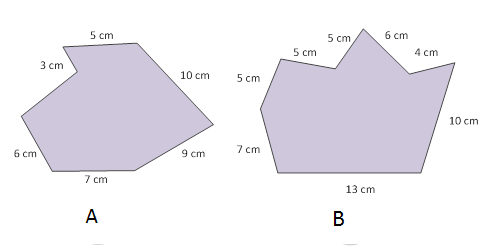### Sample Problem

Find the perimeter of the each figure.A: cm

B: cm

#### Solution

Add up the side lengths.

Perimeter of a figure A = 5+10+9+7+6+3 = 40 cm

Perimeter of a figure B = 5+5+5+6+4+10+13+7 = 55 cm